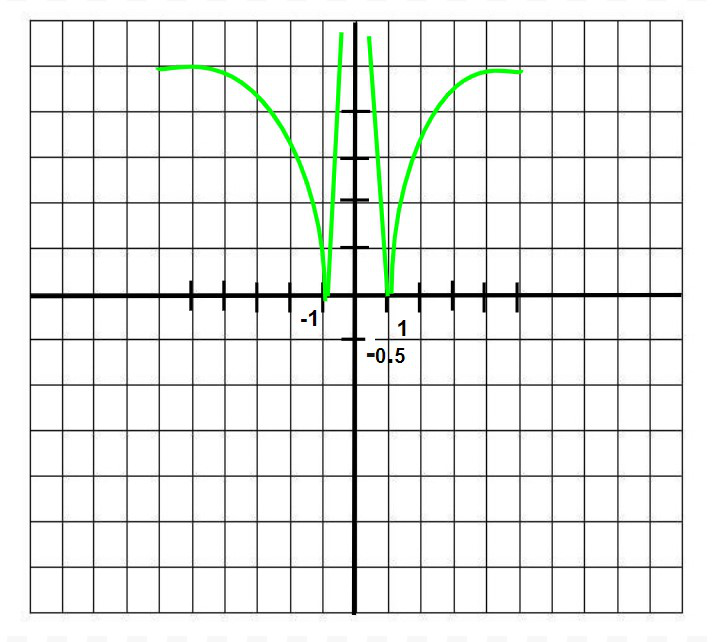Open in App
Not now

# Class 12 RD Sharma Solutions – Chapter 10 Differentiability – Exercise 10.2

• Last Updated : 16 May, 2021

### Question 1. If f is defined by f(x) = x2, find f'(2).

Solution:Hence, f'(2) = 4.

### Question 2.  If f is defined by f(x) = x2 – 4x + 7, show that f'(5) = 2f'(7/2).

Solution:f'(5) = 6                                      …….(1)f'(7/2) = 3

⇒ 2f'(7/2) = 6                           ……(2)

From (1) and (2)

f'(5) = 2f'(7/2).

### Question 3. Show that the derivative of the function f given by f(x) = 2x3 – 9x2 +12x + 9 at x = 1 and x = 2 are equal.

Solution:⇒ f'(1) = 0

Now,⇒ f'(2) = 0

Hence f'(1) = f'(2) = 0.

### Question 4. If for the function f(x) = ax2 + 7x – 4, f'(5) = 97, find a.

Solution:⇒ 97 = 10a +7

⇒ 10a = 90

⇒ a = 9

### Question 5. If f(x) = x3 + 7x2 + 8x – 9, find f'(4).

Solution:⇒ f'(4) = 112

### Question 6. Find the derivative of f(x) = mx + c at x = 0.

Solution:⇒ f'(0) = m.

### Question 7. Examine the differentiability ofSolution:

Since f(x) is a polynomial function, it is continuous and differentiable everywhere.

Differentiability at x = –2= 2= 1

Since, LHD at x = –2  ≠ RHD at x = –2

Hence f(x) is not differentiable at x = –2.

Now, Differentiability at x = 0

(LHD at x = 0)= ∞

(RHD at x = 0)= 1

Since, LHD at x = –2  ≠ RHD at x = 0

Hence f(x) is not differentiable at x = 0.

### Question 8. Write an example of a function which is everywhere continuous but fails to be differentiable at exactly five points.

Solution:

We know the modulus function f(x) = |x| is continuous but not differentiable at x = 0.

Hence, f(x) = |x| + |x – 1| + |x – 2| + |x – 3| + |x – 4| is continuous but not fails to be differentiable at x = 0,1,2,3,4.

### Question 9. Discuss the continuity and differentiability of f(x) = |log|x||.

Solution:

Graph of f(x) = |log|x||:From the graph above, it is clear that f(x) is continuous everywhere, but not differentiable at 1 and -1.

### Question 10.Discuss the continuity and differentiability of f(x) = e|x|.

Solution:For continuity:

(LHL at x = 0)= e0

= 1

(RHL at x = 0)= e0

= 1

Hence f(x) is continuous at x = 0.

For Differentiability:

(LHD at x = 0) == –1

(RHD at x = 0)= 1

Thus, f(x) is not differentiable at x = 0.

### Question 11. Discuss the differentiability ofSolution:

(LHD at x = c)= k

(RHD at x = c) == k

Clearly (LHD at x = c) = (RHD at x = c)

f(x) is differentiable at x = c.

### Question 12. Is |sinx| differentiable? What about cos|x|?

Solution:(LHD at x = nπ)= –1

(RHD at x = nπ)= 1

Since, LHD at x = nπ  ≠ RHD at x = nπ

Hence f(x) = |sinx|  is not differentiable at x = nπ.

Now, f(x) = cos|x|

Since, cos(–x) = cosx

Thus, f(x) = cos x

Hence f(x) = cos|x| is differentiable everywhere.

My Personal Notes arrow_drop_up
Related Articles